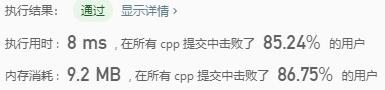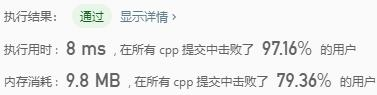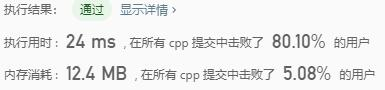# Leetcode 53：最大子序和

## 代码

int maxSubArray(vector<int>& nums) { int ThisSum = 0; int MaxSum = INT_MIN; int len = nums.size(); for (int i = 0; i < len; i++) { if (nums[i] > MaxSum) MaxSum = nums[i]; } for (int i = 0; i < len; i++) { ThisSum += nums[i]; if (ThisSum > MaxSum) MaxSum = ThisSum; else if (ThisSum < 0) ThisSum = 0; } return MaxSum;}

## 复杂度# Leetcode 55：跳跃游戏

## 代码实现

bool canJump(vector<int>& nums) {    int len = nums.size();    if (len < 1)        return false;    int lp = len - 1;    for (int i = len - 1; i >= 0; i--)    {        //倒着往回走        if (nums[i] + i >= lp) {            lp = i;        }    }    return lp == 0;}

## 复杂度# Leetcode 56：合并区间

## 代码实现

vector<vector<int>> merge(vector<vector<int>>& intervals) {    vector<vector<int>> res;    sort(intervals.begin(), intervals.end());    int cur = 0;    for (const auto& in : intervals)    {        if (res.empty()) {            res.push_back(in);        }        if (in <= res[cur]) {            if (in > res[cur])            {                res[cur] = in;            }        }        else {            res.push_back(in);            ++cur;        }    }    return res;}

## 复杂度